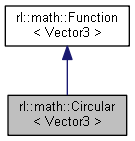Robotics Library  0.7.0
rl::math::Circular< Vector3 > Class Reference

Circular segment function that maps from a time x to a point in 3D on a circular trajectory. More...

#include <CircularVector3.h>

Inheritance diagram for rl::math::Circular< Vector3 >:[legend]

## Public Member Functions

Circular ()

virtual ~Circular ()

Circular< Vector3 > * clone () const

Real getAngle () const

Vector3 getAxisX () const

Vector3 getAxisY () const

Vector3 getCenter () const

Vector3 operator() (const Real &x, const ::std::size_t &derivative=0) const
Evaluates the circular segment function for a given x. More...Public Member Functions inherited from rl::math::Function< Vector3 >
Function ()

virtual ~Function ()

virtual Functionclone () const=0

Real duration () const

Reallower ()

const Reallower () const

Realupper ()

const Realupper () const

## Static Public Member Functions

static Circular< Vector3ThreePoints (const Vector3 &y0, const Vector3 &yi, const Vector3 &y1, const Real &x1=1)
Generates a circular segment through three given points in 3D. More...

static Circular< Vector3ThreePointsAngle (const Vector3 &y0, const Vector3 &yi, const Vector3 &y1, const Real &angle, const Real &x1=1)
Generates a circular segment through three given points in 3D with a given segment angle. More...

## Protected Attributes

Real angle
Angle of circular motion. More...

Vector3 axisX
First axis of the circular motion. More...

Vector3 axisY
Second axis of the circular motion. More...

Vector3 center
Center of the circle. More...Protected Attributes inherited from rl::math::Function< Vector3 >
Real x0

Real x1

## Detailed Description

Circular segment function that maps from a time x to a point in 3D on a circular trajectory.

## ◆ Circular()

 rl::math::Circular< Vector3 >::Circular ( )
inline

## ◆ ~Circular()

 virtual rl::math::Circular< Vector3 >::~Circular ( )
inlinevirtual

## ◆ clone()

 Circular* rl::math::Circular< Vector3 >::clone ( ) const
inline

## ◆ getAngle()

 Real rl::math::Circular< Vector3 >::getAngle ( ) const
inline

## ◆ getAxisX()

 Vector3 rl::math::Circular< Vector3 >::getAxisX ( ) const
inline

## ◆ getAxisY()

 Vector3 rl::math::Circular< Vector3 >::getAxisY ( ) const
inline

## ◆ getCenter()

 Vector3 rl::math::Circular< Vector3 >::getCenter ( ) const
inline

## ◆ operator()()

 Vector3 rl::math::Circular< Vector3 >::operator() ( const Real & x, const ::std::size_t & derivative = 0 ) const
inlinevirtual

Evaluates the circular segment function for a given x.

Note that only the first two derivatives are implemented, all higher orders will return NaN.

Implements rl::math::Function< Vector3 >.

## ◆ ThreePoints()

 static Circular rl::math::Circular< Vector3 >::ThreePoints ( const Vector3 & y0, const Vector3 & yi, const Vector3 & y1, const Real & x1 = 1 )
inlinestatic

Generates a circular segment through three given points in 3D.

The given points must not be (numerically close to) colinear.

## ◆ ThreePointsAngle()

 static Circular rl::math::Circular< Vector3 >::ThreePointsAngle ( const Vector3 & y0, const Vector3 & yi, const Vector3 & y1, const Real & angle, const Real & x1 = 1 )
inlinestatic

Generates a circular segment through three given points in 3D with a given segment angle.

The given points must not be (numerically close to) colinear. Contrary to ThreePoints, where the circular segment ends in y1, the circular segments ends after a given angle. With this, a full circle can be constructed, given an angle of 2*pi. The given segment angle may be any real number, which allows multiple rotations.

## ◆ angle

 Real rl::math::Circular< Vector3 >::angle
protected

Angle of circular motion.

Any real value is allowed.

## ◆ axisX

 Vector3 rl::math::Circular< Vector3 >::axisX
protected

First axis of the circular motion.

Note that at time 0, the function value is (center + axisX).

## ◆ axisY

 Vector3 rl::math::Circular< Vector3 >::axisY
protected

Second axis of the circular motion.

After a motion of 90 degrees, the function value is (center + axisY).

## ◆ center

 Vector3 rl::math::Circular< Vector3 >::center
protected

Center of the circle.

The documentation for this class was generated from the following file: